# Integers (Mathematics) Class 6 - NCERT Questions

Q 1.

Write opposites of the following :
(A) Increase in weight
(B) 30 km north
(C) 326 BC
(D) Loss of Rs. 700
(E) 100 m above sea level

SOLUTION:

(A) Decrease in weight
(B) 30 km south
(D) Profit of Rs. 700
(E) 100 m below sea level

Q 2.

Represent the following numbers as integers with appropriate signs.
(A) An aeroplane is flying at a height two thousand metre above the ground.
(B) A submarine is moving at a depth eight hundred metre below the sea level.
(C) A deposit of rupees two hundred.
(D) Withdrawal of rupees seven hundred.

SOLUTION:

(A) Two thousand metre above the ground = + 2000
(B) Eight hundred metre below the sea level = − 800
(C) Deposit of two hundred rupees = + 200
(D) Withdrawal of seven hundred rupees = −700

Q 3.

Represent the following numbers on a number line :
(A) + 5
(B) − 10
(C) + 8
(D) − 1
(E) − 6

SOLUTION:

(A)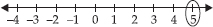(B)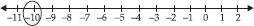(C)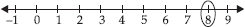(D)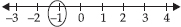(E)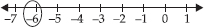Q 4.

Adjacent figure is a vertical number line, representing integers. Observe it and locate the following points :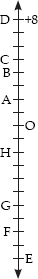(A) If point D is + 8, then which point is − 8?
(B) Is point G a negative integer or a positive integer?
(C) Write integers for points B and E.
(D) Which point marked on this number line has the least value?
(E) Arrange all the points in decreasing order of value.

SOLUTION:

(A) We have, point D is +8.
Therefore, 16 steps to the down from D is −8 i.e., the point G.
(B) Point G is a negative integer.
(C) Point B is four steps down from point D.
∴ Value of point B = +8 − 4 = +4
Point E is eighteen steps down from point D.
∴ Value of point E = +8 − 18 = −10
(D) Since, point E is located in the bottom. So, point E has the least value.
(E) Decreasing order of all the points is, D, C, B, A, O, H, G, F, E

Q 5.

Following is the list of temperatures of five places in India on a particular day of the year.

 Place Temperature Siachin 10°C below 0°C ................. Shimla 2°C below 0°C ................. Ahmedabad 30°C above 0°C ................. Delhi 20°C above 0°C ................. Srinagar 5°C below 0°C .................

(A) Write the temperatures of these places in the form of integers in the blank column.
(B) Following is the number line representing the temperature in degree Celsius.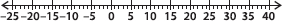Plot the name of the city against its temperature.
(C) Which is the coolest place?
(D) Write the names of the places where temperatures are above 10°C.

SOLUTION:

(A)
(B)(C) Siachin is the coolest place.
(D) Ahmedabad and Delhi have temperature above 10°C.

Q 6.

In each of the following pairs, which number is to the right of the other on the number line?
(A) 2, 9
(B) − 3, − 8
(C) 0, − 1
(D) − 11, 10
(E) − 6, 6
(F) 1, − 100

SOLUTION:

(A) 9 is right to 2
(B) −3 is right to −8
(C) 0 is right to −1
(D) 10 is right to −11
(E) 6 is right to −6
(F) 1 is right to −100

Q 7.

Write all the integers between the given pairs (write them in the increasing order.)
(A) 0 and − 7
(B) − 4 and 4
(C) − 8 and − 15
(D) − 30 and − 23

SOLUTION:

(A) The integers between 0 and −7 are −6, −5, −4, −3, −2, −1
(B) The integers between −4 and 4 are −3, −2, −1, 0, 1, 2, 3
(C) The integers between −8 and −15 are −14, −13, −12, −11, −10, −9
(D) The integers between −30 and −23 are −29, −28, −27, −26, −25, −24

Q 8.

(A) Write four negative integers greater than − 20.
(B) Write four negative integers less than − 10.

SOLUTION:

(A) There are 19 negative integers which are greater than −20. Four of them are −19, −18, −17, −16
(B) There are infinite negative integers which are less than −10. Four of them are −11, −12, −13, −14

Q 9.

For the following statements, write True (T) or False (F). If the statement is false, correct the statement.
(A) − 8 is to the right of − 10 on a number line.
(B) − 100 is to the right of − 50 on a number line.
(C) Smallest negative integer is − 1.
(D) − 26 is greater than − 25.

SOLUTION:

(A) True
(B) False : since −100 is to the left of −50 on the number line.
(C) False : since −1 is the greatest negative integer.
(D) False : since −26 is less than −25.

Q 10.

Draw a number line and answer the following :
(A) Which number will we reach if we move 4 numbers to the right of − 2.
(B) Which number will we reach if we move 5 numbers to the left of 1.
(C) If we are at − 8 on the number line, in which direction should we move to reach − 13?
(D) If we are at − 6 on the number line, in which direction should we move to reach − 1?

SOLUTION:

(A)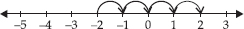Thus, we will reach 2 if we move 4 numbers to the right of −2.
(B)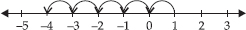Thus, we will reach − 4 if we move 5 numbers to the left of 1.
(C)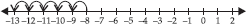Thus, we should move 5 numbers to the left of −8 to reach −13.
(D)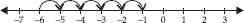Thus, we should move 5 numbers to the right of − 6 to reach −1.

Q 11.

Using the number line write the integer which is :
(A) 3 more than 5
(B) 5 more than −5
(C) 6 less than 2
(D) 3 less than −2

SOLUTION:

(A)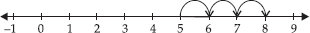Thus, 3 more than 5 is 8.
(B)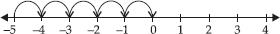Thus, 5 more than −5 is 0.
(C)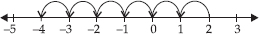Thus, 6 less than 2 is −4.
(D)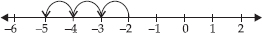Thus, 3 less than −2 is −5.

Q 12.

Use number line and add the following integers :
(A) 9 + (− 6)
(B) 5 + (− 11)
(C) (− 1) + (− 7)
(D) (− 5) + 10
(E) (− 1) + (− 2) + (− 3)
(F) (− 2) + 8 + (− 4)

SOLUTION:

(A)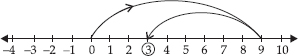Thus, 9 + (−6) = 3
(B)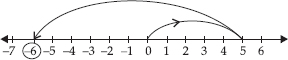Thus, 5 + (−11) = −6
(C)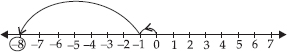Thus, (−1) + (−7) = −8
(D)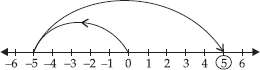Thus, (−5) + 10 = 5
(E)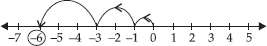Thus, (−1) + (−2) + (−3) = − 6
(F)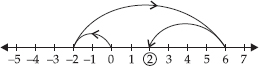Thus, (−2) + 8 + (− 4) = 2

Q 13.

Add without using number line :
(A) 11 + (− 7)
(B) (− 13) + (+ 18)
(C) (− 10) + (+ 19)
(D) (− 250) + (+ 150)
(E) (− 380) + (− 270)
(F) (− 217) + (− 100)

SOLUTION:

(A) 11 + (−7) = 11 − 7 = 4
(B) (−13) + (+18) = −13 + 18 = 5
(C) (−10) + (+19) = −10 + 19 = 9
(D) (−250) + (+150) = −250 + 150 = −100
(E) (−380) + (−270) = −380 − 270 = − 650
(F) (−217) + (−100) = −217 − 100 = −317

Q 14.

Find the sum of :
(A) 137 and − 354
(B) − 52 and 52
(C) − 312, 39 and 192
(D) − 50, − 200 and 300

SOLUTION:

(A) 137 + (−354) = 137 − 354 = −217
(B) −52 + 52 = 0
(C) −312 + 39 + 192 = − 312 + 231 = −81
(D) −50 + (−200) + 300 = −50 − 200 + 300
= − 250 + 300 = 50

Q 15.

Find the sum :
(A) (− 7) + (− 9) + 4 + 16
(B) (37) + (− 2) + (− 65) + (− 8)

SOLUTION:

(A) (−7) + (−9) + 4 + 16
= −7 − 9 + 4 + 16 = −16 + 20 = 4
(B) (37) + (−2) + (− 65) + (−8)
= 37 − 2 − 65 − 8 = 37 − 75 = − 38

Q 16.

Find
(A) 35 − (20)
(B) 72 − (90)
(C) (− 15) − (− 18)
(D) (−20) − (13)
(E) 23 − (− 12)
(F) (−32) − (− 40)

SOLUTION:

(A) 35 − 20 = 15
(B) 72 − 90 = −18
(C) (−15) − (−18) = − 15 + 18 = 3
(D) (−20) − (13) = −20 − 13 = −33
(E) 23 − (−12) = 23 + 12 = 35
(F) (−32) − (−40) = −32 + 40 = 8

Q 17.

Fill in the blanks with >, < or = sign.
(A) (− 3) + (− 6) ______ (− 3) − (− 6)
(B) (− 21) − (− 10) _____ (− 31) + (− 11)
(C) 45 − (− 11) ______ 57 + (− 4)
(D) (− 25) − (− 42) _____ (− 42) − (− 25)

SOLUTION:

(A) < : (−3) + (−6) = −3 − 6 = −9
(−3) − (−6) = −3 + 6 = 3
Since, −9 < 3
∴ (−3) + (−6) < (−3) − (−6)
(B) > : (−21) − (−10) = −21 + 10 = −11
(−31) + (−11) = −31 − 11 = − 42
Since, −11 > − 42
∴ (−21) − (−10) > (−31) + (−11)
(C) > : 45 − (−11) = 45 + 11 = 56
57 + (−4) = 57 − 4 = 53
Since, 56 > 53
∴ 45 − (−11) > 57 + (−4)
(D) > : (−25) − (−42) = −25 + 42 = 17
(−42) − (−25) = −42 + 25 = −17
Since, 17 > −17
∴ (−25) − (−42) > (− 42) − (−25)

Q 18.

Fill in the blanks.
(A) (− 8) + _____ = 0
(B) 13 + _____ = 0
(C) 12 + (− 12) = ____
(D) (− 4) + ____ = − 12
(E) ____ − 15 = − 10

SOLUTION:

(A) 8 : (−8) + 8 = 0
(B) −13 : 13 + (−13) = 0
(C) 0 : 12 + (−12) = 0
(D) −8 : (−4) + (−8) = − 12
(E) 5 : 5 − 15 = −10

Q 19.

Find
(A) (− 7) − 8 − (− 25)
(B) (− 13) + 32 − 8 − 1
(C) (− 7) + (− 8) + (− 90)
(D) 50 − (− 40) − (− 2)

SOLUTION:

(A) (−7) − 8 − (−25)
= −7 − 8 + 25 = −15 + 25 = 10
(B) (−13) + 32 − 8 − 1
= −13 + 32 − 8 − 1 = 32 − 22 = 10
(C) (−7) + (−8) + (−90)
= −7 − 8 − 90 = − 105
(D) 50 − (−40) − (−2)
= 50 + 40 + 2 = 92